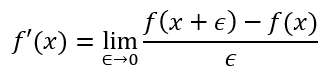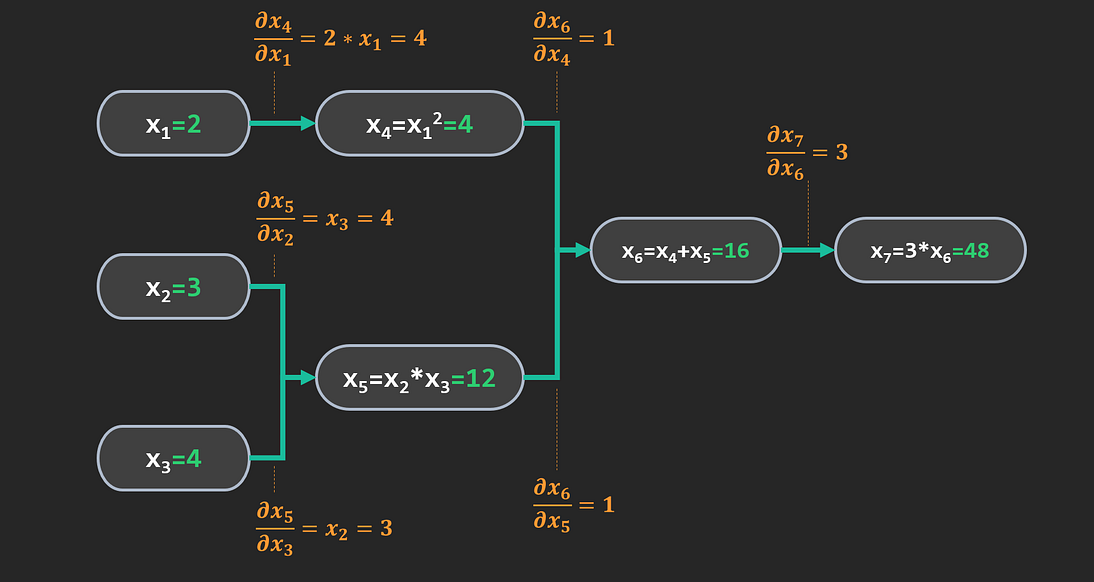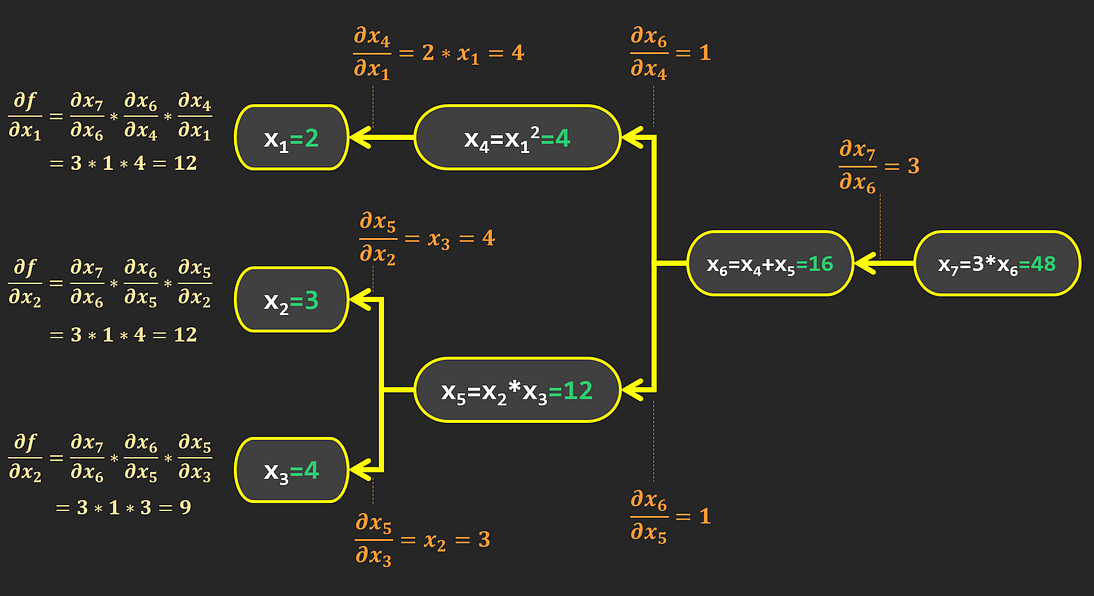2021-08-03
###### What are eigenvectors and eigenvalues?
2021-08-06

Introduction

There are several methods to calculate gradients in computer programs: (1) Manual differentiation; (2) Symbolic differentiation; (3) Finite differences approximation; and (4) Automatic differentiation, which we will share further details in this post.

Let’s start with a practical example: suppose that we have the following function f, which accepts 3 arguments:

Suppose we want to find the partial derivatives of f in a given point (x1=2, x2=3, x3=4). Let’s examine how this is done for each method.

# 1. Manual differentiation

With manual differentiation, we need to figure out the derivative of ourselves using calculus, and implement it in our program. In this example we can work out the derivative using these simple rules from calculus:

• constant factor rule: for aR :
• polynomial rule: for nR:
• linearity: for any functions f and ga and bR:

This gives the following values for our partial derivatives, evaluated at point (x1=2, x2=3, x3=4):

This process works in our example, as the function f is simple; it is still manual though, and might become cumbersome for more complex functions. The good news is that it can be automated via symbolic differentiation.

# 2. Symbolic differentiation

In symbolic differentiation, the mathematical expression of the function is parsed and converted into elementary computation nodes. These elementary blocks correspond to basic functions for which derivatives are easily expressed (scalar product, polynomials, exponential, logarithm, sine, and cosine… etc.).

The derivatives of these elementary blocks are then assembled using the rules for combined functions (linearity, product, inverse, and compound rules), to obtain the final form of f’(x).

Libraries such as sympy in python can perform symbolic differentiation:https://medium.com/media/07afbd483f1e35ef83163e92c7dfd7a5

However, for complex functions, the graph obtained can become very big. Pruning is possible, but it is quite tricky.

In practice this can result in slow execution when evaluating the values of the derivatives, leading to sub-optimal performance.

# 3. Finite differences approximation

Finite difference approximation makes use of the following definition for the derivative of f in x:Limit of Newton’s Difference Quotient formula

If we choose a small value for epsilon, we can evaluate f(x+epsilon) and f(x), and calculate a local approximation for f’(x) using the formula above. The smaller epsilon, the better the approximation. This method is very robust and can be used for any arbitrary function f.

However, the result is an approximation and can be inexact if the function is non-linear. For example, in our case f depends on the square of x1, which is not linear. To visualize the approximation error, let’s plot the partial derivative of f with respect to x1 when the bump size epsilon varies (note: for convenience, we express epsilon in % of x1):

As you can see the error varies with epsilon, hence our results will depend on our choice of this value.

But the major limitation of this method is computational rather than numerical. You might have noticed that calculating df/dx1 required evaluating f twice: once for f(x1, x2, x3) and once for f(x1*(1+epsilon), x2, x3).

Similarly, for a function of 100,000 variables you would need to evaluate f 100,001 times: once in the original point, and once after bumping each individual parameter. This would be computationally expensive.

If our function f was a Deep Neural Network with millions of parameters, evaluating each partial derivative this way would be very time-consuming.

Fortunately, there is another more efficient approach: Autodiff 😎.

# 4. Automatic Differentiation

Autodiff is an elegant approach that can be used to calculate the partial derivatives of any arbitrary function in a given point. It decomposes the function in a sequence of elementary arithmetic operations (+, -, *, /) and functions (max, exp, log, cos, sin…); then uses the chain rule to work out the function’s derivative with respect to its initial parameters.

Note: there are 2 variants of Autodiff:

• Forward-Mode, which is a hybrid of symbolic and numerical differentiation; while numerically precise, it requires one pass through the computational graph for each input parameter, which is resource consuming.
• Reverse-Mode, on the other hand, only requires 2 passes through the computational graph to evaluate both the function and its partial derivatives.

In this post we focus on Reverse-Mode Autodiff, as it is the most popular in practical implementations; for example it is the one used in Tensorflow.

To see how this magic works, let’s start by representing the function f as a computational graph:

There are 2 steps to Reverse-Mode autodiff: a forward pass, during which the function value at the selected point is calculated; and a backward pass, during which the partial derivatives are evaluated.

Forward pass

During the forward pass, the function inputs are propagated down the computational graph:

As expected we get the function value: f(2, 3, 4)=48. We also assigned names to intermediate nodes encountered along the way: x4x5x6x7; they will be used below.

Now let’s calculate the gradients.

Backward pass

Reverse-Mode autodiff uses the chain rule to calculate the gradient values at point (2, 3, 4).

Let’s calculate the partial derivatives of each node with respect to its immediate inputs:Partial derivatives of each node with respect to its inputs

Note that we can calculate the numerical value of each partial derivative — for example, dx5/dx3=x2=3 — thanks to the value for x2 obtained during the forward pass. Also note that the partial derivatives are calculated locally at point (2, 3, 4); should we change the initial point, the values of the derivatives would also change.

Now that we have the partial derivatives of each node, we can use the chain rule to calculate the partial derivatives of f with respect to its original inputs: x1x2, and x3.

In calculus, the chain rule is a formula for computing the derivative of the composition of two or more functions:

Remember that we are interested in the following gradient values, evaluated at point (2, 3, 4):

By traversing the graph from right to left, we can express the partial derivative of with respect to x1 as follow:

Similarly, we calculate the partial derivatives with respect to x2 and x3:

Finally, we get:Final values of partial derivatives obtained after the backward pass

In the end, we obtain the same results as manual and symbolic differentiation:

• df/dx1=12
• df/dx2=12
• df/dx3=9

The chain rule has an intuitive effect: the sensitivity of (or x7) with respect to an input, say x1, is the product of the sensitivities of each node encountered along the way from x1 to x7: the sensitivities “propagate” down the computational graph.

Taking the same example of df/dx1=12, we see that this value is due mostly to the sensitivity of x4 with respect to x1 (*4), and the sensitivity to x7 with respect to x6 (*3).

# A practical application: Gradient Descent

In Deep Learning, Neural Networks are usually trained using Gradient Descent. We won’t go into details on this topic here, but rather illustrate how Autodiff is used in this context.

Using our example as an analogy: x1x2, and x3 would be the Neural Network parameters (that we want to determine), and x7 would be an error or cost function (that we want to minimize).

Our initial weights are (x1=2, x2=3, x3=4) which gives a “cost” of 48. We then calculate the partial derivatives of with autodiff, and obtain (12, 12, 9).

Observe that all 3 derivatives are positive; for example, if we increase x1 by 1, our “cost” will increase by 12. Furthermore, f is positive (worth 48), so increasing x1 would make more positive. But we want to minimize f, so we need to decrease x1:

In practice, cost functions are usually designed to be positive, for example, the L2 distance between 2 vectors of “predictions” and “target” values. Each iteration of gradient descent will adjust the weights simultaneously by taking a “baby step” along the negative gradient direction.

# Conclusion

This article presented several methods of computational differentiation, with a focus on reverse-mode autodiff.

https://medium.com/@rhome/automatic-differentiation-26d5a993692b##### Amir Masoud Sefidian
Machine Learning Engineer